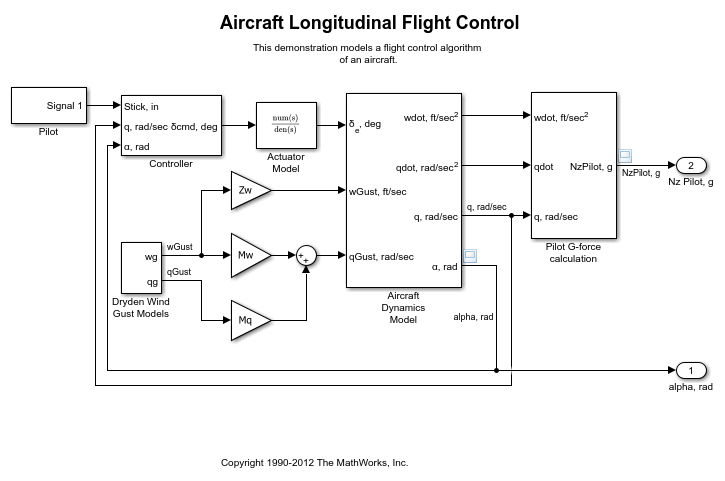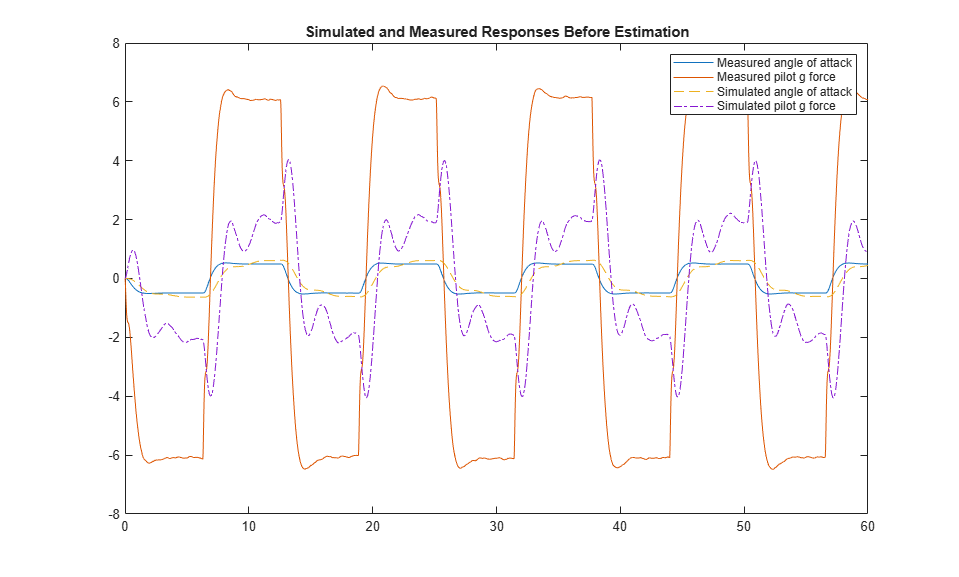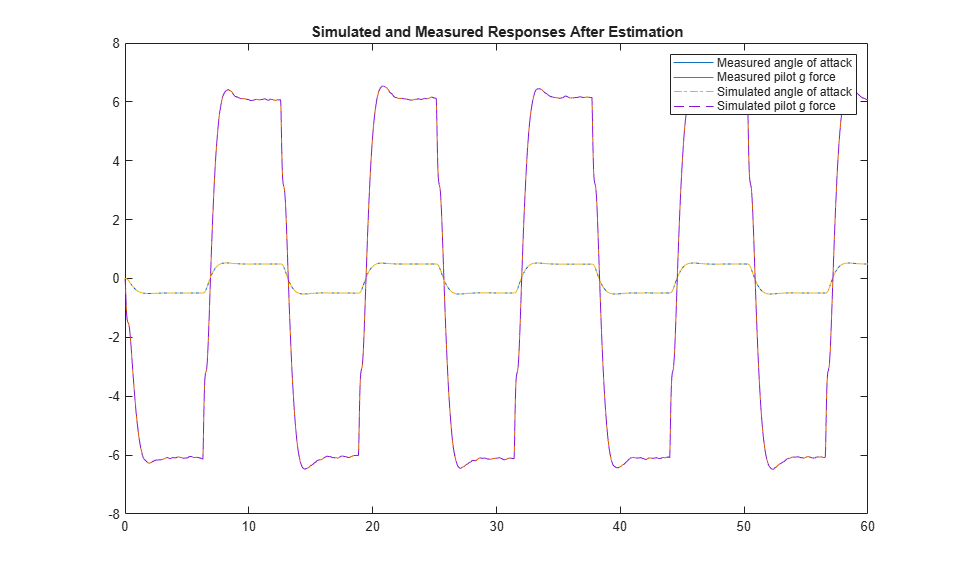# Estimate Model Parameter Values (Code)

This example shows how to use experimental data to estimate model parameter values.

### Aircraft Model

The Simulink® model, `sdoAircraftEstimation`, models the longitudinal flight control system of an aircraft.

```open_system('sdoAircraftEstimation') ```### Estimation Problem

You use measured data to estimate the aircraft model parameters and states.

Measured output data:

• Pilot G force, output of the Pilot G-force calculation block

• Angle of attack, fourth output of the Aircraft Dynamics Model block

Parameters:

• Actuator time constant, `Ta`, used by the Actuator Model block

• Vertical velocity, `Zd`, used by the Aircraft Dynamics Model block

• Pitch rate gains, `Md`, used by the Aircraft Dynamics Model block

State:

• Initial state of the first-order actuator model, sdoAircraftEstimation/Actuator Model

### Define the Estimation Experiment

Get the measured data.

```[time,iodata] = sdoAircraftEstimation_Experiment; ```

The `sdoAircraftEstimation_Experiment` function returns the measured output data, `iodata`, and the corresponding time vector. The first column of `iodata` is the pilot G force and the second column is the angle of attack.

To see the code for this function, type `edit sdoAircraftEstimation_Experiment`.

Create an experiment object to store the measured input/output data.

```Exp = sdo.Experiment('sdoAircraftEstimation'); ```

Create an object to store the measured pilot G-Force output.

```PilotG = Simulink.SimulationData.Signal; PilotG.Name = 'PilotG'; PilotG.BlockPath = 'sdoAircraftEstimation/Pilot G-force calculation'; PilotG.PortType = 'outport'; PilotG.PortIndex = 1; PilotG.Values = timeseries(iodata(:,2),time); ```

Create an object to store the measured angle of attack (alpha) output.

```AoA = Simulink.SimulationData.Signal; AoA.Name = 'AngleOfAttack'; AoA.BlockPath = 'sdoAircraftEstimation/Aircraft Dynamics Model'; AoA.PortType = 'outport'; AoA.PortIndex = 4; AoA.Values = timeseries(iodata(:,1),time); ```

Add the measured pilot G-Force and angle of attack data to the experiment as the expected output data.

```Exp.OutputData = [... PilotG; ... AoA]; ```

Add the initial state for the Actuator Model block to the experiment. Set its `Free` field to `true` so that it is estimated.

```Exp.InitialStates = sdo.getStateFromModel('sdoAircraftEstimation','Actuator Model'); Exp.InitialStates.Minimum = 0; Exp.InitialStates.Free = true; ```

### Compare the Measured Output and the Initial Simulated Output

Create a simulation scenario using the experiment and obtain the simulated output.

```Simulator = createSimulator(Exp); Simulator = sim(Simulator); ```

Search for the pilot G-Force and angle of attack signals in the logged simulation data.

```SimLog = find(Simulator.LoggedData,get_param('sdoAircraftEstimation','SignalLoggingName')); PilotGSignal = find(SimLog,'PilotG'); AoASignal = find(SimLog,'AngleOfAttack'); ```

Plot the measured and simulated data.

As expected, the model response does not match the experimental output data.

```plot(time, iodata, ... AoASignal.Values.Time,AoASignal.Values.Data,'--', ... PilotGSignal.Values.Time,PilotGSignal.Values.Data,'-.'); title('Simulated and Measured Responses Before Estimation') legend('Measured angle of attack', 'Measured pilot g force', ... 'Simulated angle of attack', 'Simulated pilot g force'); ```### Specify the Parameters to Estimate

Select the model parameters that describe the flight control actuation system. Specify bounds for the estimated parameter values based on the understanding of the actuation system.

```p = sdo.getParameterFromModel('sdoAircraftEstimation',{'Ta','Md','Zd'}); p(1).Minimum = 0.01; %Ta p(1).Maximum = 1; p(2).Minimum = -10; %Md p(2).Maximum = 0; p(3).Minimum = -100; %Zd p(3).Maximum = 0; ```

Get the actuator initial state value that is to be estimated from the experiment.

```s = getValuesToEstimate(Exp); ```

Group the model parameters and initial states to be estimated together.

```v = [p;s] ```
``` v(1,1) = Name: 'Ta' Value: 0.5000 Minimum: 0.0100 Maximum: 1 Free: 1 Scale: 0.5000 Info: [1x1 struct] v(2,1) = Name: 'Md' Value: -1 Minimum: -10 Maximum: 0 Free: 1 Scale: 1 Info: [1x1 struct] v(3,1) = Name: 'Zd' Value: -80 Minimum: -100 Maximum: 0 Free: 1 Scale: 128 Info: [1x1 struct] v(4,1) = Name: 'sdoAircraftEstimation/Actuator...' Value: 0 Minimum: 0 Maximum: Inf Free: 1 Scale: 1 dxValue: 0 dxFree: 1 Info: [1x1 struct] 4x1 param.Continuous ```

### Define the Estimation Objective Function

Create an estimation objective function to evaluate how closely the simulation output, generated using the estimated parameter values, matches the measured data.

Use an anonymous function with one input argument that calls the `sdoAircraftEstimation_Objective` function. Pass the anonymous function to `sdo.optimize`, which evaluates the function at each optimization iteration.

```estFcn = @(v) sdoAircraftEstimation_Objective(v,Simulator,Exp); ```

The `sdoAircraftEstimation_Objective` function:

• Has one input argument that specifies the actuator parameter values and the actuator initial state.

• Has one input argument that specifies the experiment object containing the measured data.

• Returns a vector of errors between simulated and experimental outputs.

The `sdoAircraftEstimation_Objective` function requires two inputs, but `sdo.optimize` requires a function with one input argument. To work around this, `estFcn` is an anonymous function with one input argument, `v`, but it calls `sdoAircraftEstimation_Objective` using two input arguments, `v` and `Exp`.

For more information regarding anonymous functions, see Anonymous Functions.

The `sdo.optimize` command minimizes the return argument of the anonymous function `estFcn`, that is, the residual errors returned by `sdoAircraftEstimation_Objective`. For more details on how to write an objective/constraint function to use with the `sdo.optimize` command, type `help sdoExampleCostFunction` at the MATLAB® command prompt.

To examine the estimation objective function in more detail, type `edit sdoAircraftEstimation_Objective` at the MATLAB command prompt.

```type sdoAircraftEstimation_Objective ```
```function vals = sdoAircraftEstimation_Objective(v,Simulator,Exp) %SDOAIRCRAFTESTIMATION_OBJECTIVE % % The sdoAircraftEstimation_Objective function is used to compare model % outputs against experimental data. % % vals = sdoAircraftEstimation_Objective(v,Exp) % % The |v| input argument is a vector of estimated model parameter values % and initial states. % % The |Simulator| input argument is a simulation object used % simulate the model with the estimated parameter values. % % The |Exp| input argument contains the estimation experiment data. % % The |vals| return argument contains information about how well the % model simulation results match the experimental data and is used by % the |sdo.optimize| function to estimate the model parameters. % % See also sdo.optimize, sdoExampleCostFunction, % sdoAircraftEstimation_cmddemo % % Copyright 2012-2015 The MathWorks, Inc. %% % Define a signal tracking requirement to compute how well the model output % matches the experiment data. Configure the tracking requirement so that % it returns the tracking error residuals (rather than the % sum-squared-error) and does not normalize the errors. % r = sdo.requirements.SignalTracking; r.Type = '=='; r.Method = 'Residuals'; r.Normalize = 'off'; %% % Update the experiments with the estimated parameter values. % Exp = setEstimatedValues(Exp,v); %% % Simulate the model and compare model outputs with measured experiment % data. % Simulator = createSimulator(Exp,Simulator); Simulator = sim(Simulator); SimLog = find(Simulator.LoggedData,get_param('sdoAircraftEstimation','SignalLoggingName')); PilotGSignal = find(SimLog,'PilotG'); AoASignal = find(SimLog,'AngleOfAttack'); PilotGError = evalRequirement(r,PilotGSignal.Values,Exp.OutputData(1).Values); AoAError = evalRequirement(r,AoASignal.Values,Exp.OutputData(2).Values); %% % Return the residual errors to the optimization solver. % vals.F = [PilotGError(:); AoAError(:)]; end ```

### Estimate the Parameters

Use the `sdo.optimize` function to estimate the actuator parameter values and initial state.

Specify the optimization options. The estimation function `sdoAircraftEstimation_Objective` returns the error residuals between simulated and experimental data and does not include any constraints, making this problem ideal for the `lsqnonlin` solver.

```opt = sdo.OptimizeOptions; opt.Method = 'lsqnonlin'; ```

Estimate the parameters.

```vOpt = sdo.optimize(estFcn,v,opt) ```
``` Optimization started 31-Aug-2022 08:13:01 First-order Iter F-count f(x) Step-size optimality 0 8 27955.2 1 1 17 10121.6 0.4744 5.68e+04 2 26 3127.27 0.3854 1.24e+04 3 35 872.666 0.4288 2.81e+03 4 44 238.616 0.5154 617 5 53 71.693 0.4938 148 6 62 17.1565 0.4236 43.9 7 71 1.73233 0.3027 11 8 80 0.0392376 0.135 1.33 9 89 0.000957796 0.02489 0.266 10 98 0.000202147 0.007931 0.0114 Local minimum possible. lsqnonlin stopped because the final change in the sum of squares relative to its initial value is less than the value of the function tolerance. vOpt(1,1) = Name: 'Ta' Value: 0.0500 Minimum: 0.0100 Maximum: 1 Free: 1 Scale: 0.5000 Info: [1x1 struct] vOpt(2,1) = Name: 'Md' Value: -6.8849 Minimum: -10 Maximum: 0 Free: 1 Scale: 1 Info: [1x1 struct] vOpt(3,1) = Name: 'Zd' Value: -63.9989 Minimum: -100 Maximum: 0 Free: 1 Scale: 128 Info: [1x1 struct] vOpt(4,1) = Name: 'sdoAircraftEstimation/Actuator...' Value: 1.1976e-04 Minimum: 0 Maximum: Inf Free: 1 Scale: 1 dxValue: 0 dxFree: 1 Info: [1x1 struct] 4x1 param.Continuous ```

### Compare the Measured Output and the Final Simulated Output

Update the experiments with the estimated parameter values.

```Exp = setEstimatedValues(Exp,vOpt); ```

Simulate the model using the updated experiment and compare the simulated output with the experimental data.

The model response using the estimated parameter values closely matches the experiment output data.

```Simulator = createSimulator(Exp,Simulator); Simulator = sim(Simulator); SimLog = find(Simulator.LoggedData,get_param('sdoAircraftEstimation','SignalLoggingName')); PilotGSignal = find(SimLog,'PilotG'); AoASignal = find(SimLog,'AngleOfAttack'); plot(time, iodata, ... AoASignal.Values.Time,AoASignal.Values.Data,'-.', ... PilotGSignal.Values.Time,PilotGSignal.Values.Data,'--') title('Simulated and Measured Responses After Estimation') legend('Measured angle of attack', 'Measured pilot g force', ... 'Simulated angle of attack', 'Simulated pilot g force'); ```### Update the Model Parameter Values

Update the model with the estimated actuator parameter values. Do not update the model actuator initial state (fourth element of `vOpt`) as it is dependent on the experiment.

```sdo.setValueInModel('sdoAircraftEstimation',vOpt(1:3)); ```

### Related Examples

To learn how to estimate model parameters using the Parameter Estimator app, see Estimate Model Parameter Values (GUI).

Close the model.

```bdclose('sdoAircraftEstimation') ```